Sphere VS

Find the surface and volume of a sphere that has a radius of 2 dm.

Result

S =  50.265 dm2
V =  33.51 dm3

Solution:Leave us a comment of example and its solution (i.e. if it is still somewhat unclear...):Be the first to comment!To solve this example are needed these knowledge from mathematics:

Tip: Our volume units converter will help you with converion of volume units.

Next similar examples:

1. Volume of ballFind the volume of a volleyball that has a radius of 4 1/2 decimeters. Use 22/7 for π
2. Spheres in sphere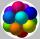How many spheres with a radius of 15 cm can fits into the larger sphere with a radius of 150 cm?
3. Observatory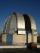Observatory dome has the shape of a hemisphere with a diameter d = 10 m. Calculate the surface.
4. Fit ballWhat is the size of the surface of Gymball (FIT - ball) with a diameter of 65 cm?
5. InscribedCube is inscribed in the cube. Determine its volume if the edge of the cube is 10 cm long.
6. Shots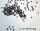5500 lead shots with diameter 4 mm is decanted into a ball. What is it diameter?
7. GasholderThe gasholder has spherical shape with a diameter 20 m. How many m3 can hold in?
8. Cube wallsFind the volume and the surface area of the cube if the area of one of its walls is 40 cm2.
9. Cuboid - simple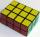Calculate the surface area and volume of a cuboid if a = 8 cm, b = 14 cm and c = 6 cm.
10. The volumeThe volume of a solid cylinder is 260 cm3 the cylinder is melt down into a cuboid, whose base is a square of 5cm, calculate the height of the cuboid and the surface area of the cuboid
11. GreenhouseGarden plastic greenhouse is shaped half cylinder with a diameter of 6 m and base length 20 m. At least how many m2 of plastic is need to its cover?
12. KitchenKitchen roller has a diameter 70 mm and width of 359 mm. How many square millimeters roll on one turn?
13. Truncated coneCalculate the volume of a truncated cone with base radiuses r1=13 cm, r2 = 10 cm and height v = 8 cm.
14. Total displacementCalculate total displacement of the 4-cylinder engine with the diameter of the piston bore B = 6.6 cm and stroke S=2.4 cm of the piston. Help: the crankshaft makes one revolution while the piston moves from the top of the cylinder to the bottom and back.
15. Common cylinderI've quite common example of a rotary cylinder. Known: S1 = 1 m2, r = 0.1 m Calculate : v =? V =? You can verify the results?
16. Milk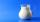At the kindergarten, every child got 1/5 liter of milk in the morning and another 1/8 liter of milk in the afternoon. How many liters were consumed per day for 20 children?
17. Base, percents, valueBase is 344084 which is 100 %. How many percent is 384177?